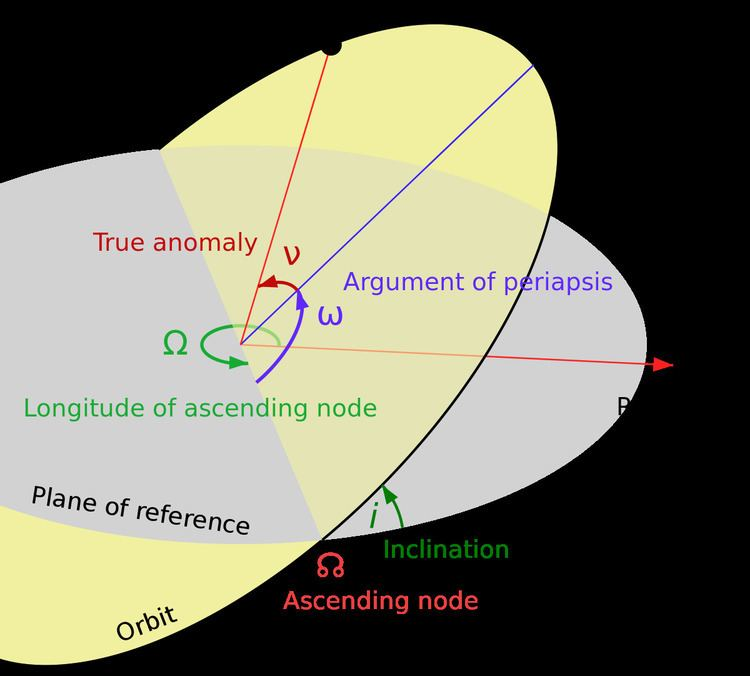# Orbital inclination

Updated on
Edit
Like
CommentOrbital inclination measures the tilt of an object's orbit around a celestial body. It is expressed as the angle between a reference plane and the orbital plane or axis of direction of the orbiting object.

## Contents

For a satellite orbiting the Earth directly above the equator, the plane of the satellite's orbit is the same as the Earth's equatorial plane, and the satellite's orbital inclination is 0°. The general case is that the satellite's orbit is tilted; it spends half an orbit over the northern hemisphere and half over the southern. If the orbit swung between 20° north latitude and 20° south latitude, then its orbital inclination would be 20°.

## Orbits

The inclination is one of the six orbital elements describing the shape and orientation of a celestial orbit. It is the angle between the orbital plane and the plane of reference, normally stated in degrees. For a satellite orbiting a planet, the plane of reference is usually the plane containing the planet's equator. For planets in the Solar System, the plane of reference is usually the ecliptic, the plane in which the Earth orbits the Sun. This reference plane is most practical for Earth-based observers. Therefore, Earth's inclination is, by definition, zero.

Inclination could instead be measured with respect to another plane, such as the Sun's equator or the invariable plane (the plane that represents the angular momentum of the Solar System, approximately the orbital plane of Jupiter).

Most planetary orbits in the Solar System have relatively small inclinations, both in relation to each other and to the Sun's equator:

On the other hand, the dwarf planets Pluto and Eris have inclinations to the ecliptic of 17° and 44° respectively, and the large asteroid Pallas is inclined at 34°.

## Natural and artificial satellites

The inclination of orbits of natural or artificial satellites is measured relative to the equatorial plane of the body they orbit, if they orbit sufficiently closely. The equatorial plane is the plane perpendicular to the axis of rotation of the central body.

An inclination of 30° could also be described using an angle of 150°. The convention is that the normal orbit is prograde, an orbit in the same direction as the planet rotates. Inclinations greater than 90° describe retrograde orbits. Thus:

• An inclination of 0° means the orbiting body has a prograde orbit in the planet's equatorial plane.
• An inclination greater than 0° and less than 90° also describe prograde orbits.
• An inclination of 63.4° is often called a critical inclination, when describing artificial satellites orbiting the Earth, because they have zero apogee drift.
• An inclination of exactly 90° is a polar orbit, in which the spacecraft passes over the north and south poles of the planet.
• An inclination greater than 90° and less than 180° is a retrograde orbit.
• An inclination of exactly 180° is a retrograde equatorial orbit.
• For impact-generated moons of terrestrial planets not too far from their star, with a large planet–moon distance, the orbital planes of moons tend to be aligned with the planet's orbit around the star due to tides from the star, but if the planet–moon distance is small, it may be inclined. For gas giants, the orbits of moons tend to be aligned with the giant planet's equator, because these formed in circumplanetary disks.

## Exoplanets and multiple star systems

The inclination of exoplanets or members of multiple stars is the angle of the plane of the orbit relative to the plane perpendicular to the line-of-sight from Earth to the object.

• An inclination of 0° is a face-on orbit, meaning the plane of its orbit is parallel to the sky.
• An inclination of 90° is an edge-on orbit, meaning the plane of its orbit is perpendicular to the sky.
• Since the word 'inclination' is used in exoplanet studies for this line-of-sight inclination then the angle between the planet's orbit and the star's rotation must use a different word and is termed the spin-orbit angle or spin-orbit alignment. In most cases the orientation of the star's rotational axis is unknown.

Because the radial-velocity method more easily finds planets with orbits closer to edge-on, most exoplanets found by this method have inclinations between 45° and 135°, although in most cases the inclination is not known. Consequently, most exoplanets found by radial velocity have true masses no more than 70% greater than their minimum masses. If the orbit is almost face-on, especially for superjovians detected by radial velocity, then those objects may actually be brown dwarfs or even red dwarfs. One particular example is HD 33636 B, which has true mass 142 MJ, corresponding to an M6V star, while its minimum mass was 9.28 MJ.

If the orbit is almost edge-on, then the planet can be seen transiting its star.

## Other meaning

For planets and other rotating celestial bodies, the angle of the equatorial plane relative to the orbital plane — such as the tilt of the Earth's poles toward or away from the Sun — is sometimes also called inclination, but less ambiguous terms are axial tilt or obliquity.

## Calculation

In astrodynamics, the inclination i can be computed from the orbital momentum vector h (or any vector perpendicular to the orbital plane) as

i = arccos h z | h | ,

where hz is the z-component of h.

Mutual inclination of two orbits may be calculated from their inclinations to another plane using cosine rule for angles.

Orbital inclination Wikipedia